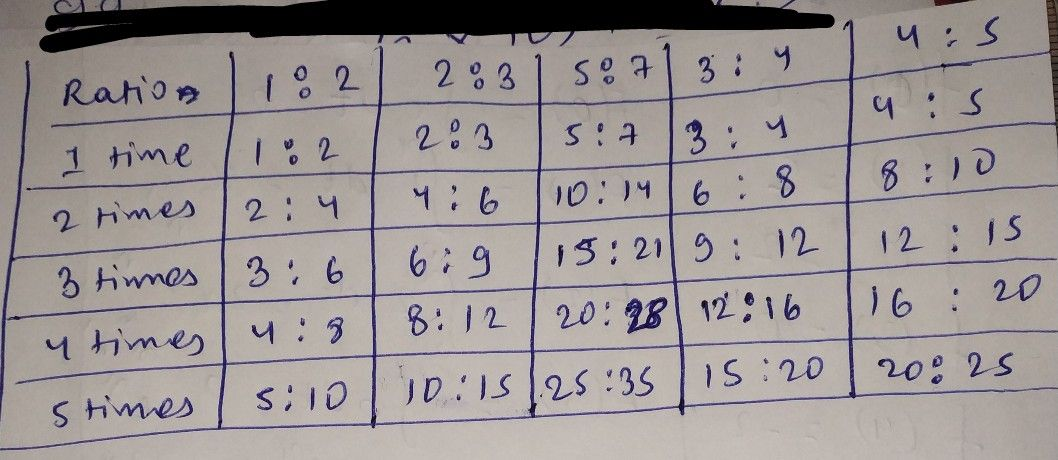Symbol
ProblemComplete the following tables Ratio $1.2$ $2.3$ $5:.7$ $1$ time $1:2$ $1$ $2$ times $4:6$ $.$ $3$ times $15.21$ 65 $12:16$ 4times $20.25$ $5$ times
10th-13th grade
Other
SolutionQanda teacher - rosh
hereif you have any doubt please feel free to askStudent
I got it, thanks you!!